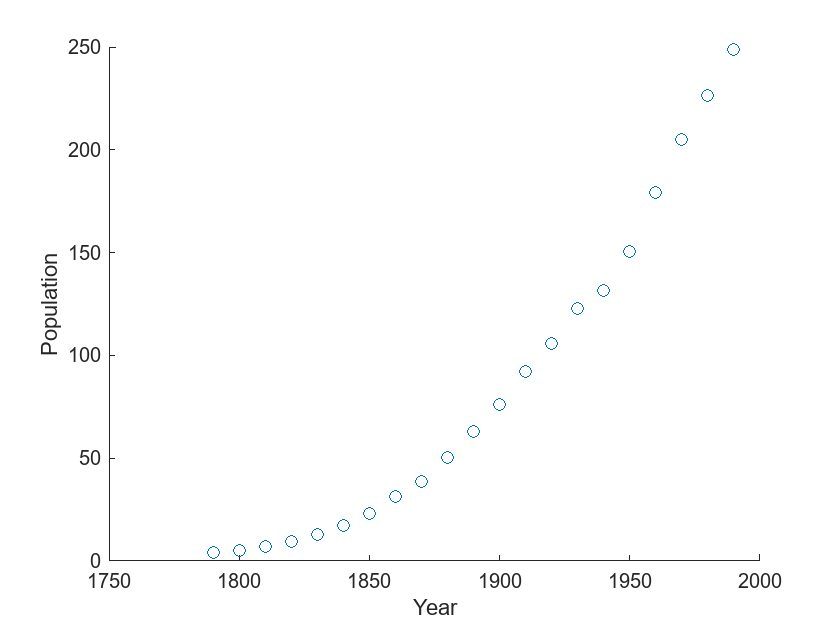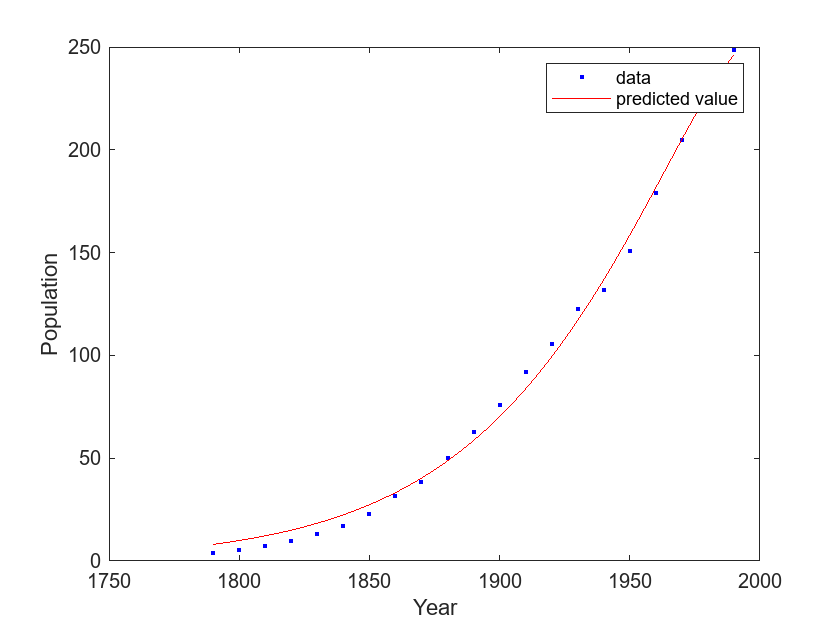# Fit Exponential Model to Data

This example shows how to fit an exponential model to data using the trust-region and Levenberg-Marquardt nonlinear least-squares algorithms.

Load the `census` data set.

`load census`

The variables `pop` and `cdate` contain data for the population size and the year the census was taken, respectively.

Display a scatter plot of the data.

```scatter(cdate,pop) xlabel("Year") ylabel("Population")```The plot shows that the population increases from year to year in a shape that resembles an exponential function.

Fit a two-term exponential model to the population data using the default trust-region fitting algorithm. Return the results of the fit and the goodness-of-fit statistics.

`[exp_tr,gof_tr] = fit(cdate,pop,"exp2")`
```exp_tr = General model Exp2: exp_tr(x) = a*exp(b*x) + c*exp(d*x) Coefficients (with 95% confidence bounds): a = 7.169e-17 b = 0.02155 c = 0 d = 0.02155 ```
```gof_tr = struct with fields: sse: 1.2412e+04 rsquare: 0.8995 dfe: 17 adjrsquare: 0.8818 rmse: 27.0209 ```

`exp_tr` contains the results of the fit, including coefficients calculated with the trust-region fitting algorithm. The goodness-of-fit statistics stored in `gof_tr` include the root mean squared error (RMSE) of 27.0209.

Plot the model in `exp_tr` together with a scatter plot of the data.

```plot(exp_tr,cdate,pop) legend(["data","predicted value"]) xlabel("Year") ylabel("Population")```The plot shows that the model in `exp_tr` does not closely follow the census data.

Improve the fit by using the Levenberg-Marquardt fitting algorithm to calculate the coefficients.

`[exp_lm,gof_lm] = fit(cdate,pop,"exp2",Algorithm="Levenberg-Marquardt")`
```exp_lm = General model Exp2: exp_lm(x) = a*exp(b*x) + c*exp(d*x) Coefficients (with 95% confidence bounds): a = 4.282e-17 (-1.125e-11, 1.126e-11) b = 0.02477 (-5.67, 5.719) c = -3.933e-17 (-1.126e-11, 1.126e-11) d = 0.02481 (-5.696, 5.745) ```
```gof_lm = struct with fields: sse: 475.9498 rsquare: 0.9961 dfe: 17 adjrsquare: 0.9955 rmse: 5.2912 ```

`exp_lm` contains the results of the fit, including coefficients calculated with the Levenberg-Marquardt fitting algorithm. The goodness-of-fit statistics stored in `gof_lm` include the RMSE of 5.2912, which is smaller than the RMSE for `exp_tr`. The relative sizes of the RMSEs indicate that the model stored in `exp_lm` fits the data more accurately than the model stored in `exp_tr`.

Plot the model in `exp_lm` together with a scatter plot of the data.

```plot(exp_lm,cdate,pop) legend(["data","predicted value"]) xlabel("Year") ylabel("Population")```The plot shows that the model in `exp_lm` follows the census data more closely than the model in `exp_tr`.